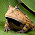### Write .ods (for LibreOffice Calc) from Python (2.7) (Ubuntu 12.10)

--------------------------------

Edit 10-02-2015

The Simple ods py module doesn't seem to work with updated versions of odfpy. Testing with simpleodspy 1.1 and odfpy 1.3.1 throws a failed assertion, while simpleodspy-1.1 and odfpy 0.9.6 works.

It doesn't look like simpleodspy is being maintained any longer. The best bet may be to use odfpy directly if you can't get your hands on an older version of odfpy.

-------------------------------

I was looking for an easy way to write .ods files (for LibreOffice Calc) from Python (2.7). I really only needed the functionality of designating the contents of a cell and changing the background color of a cell based on its contents.

Simple ods py, written by Yaacov Zamir, worked very well for this purpose. It required the odfpy module for the functionality I needed. Here's what worked for me:

https://pypi.python.org/pypi/odfpy

http://simple-odspy.sourceforge.net/

```python setup.py build
sudo python setup.py install
```
This delightful post on Stack Overflow has a very nice way of getting the string corresponding to a column index in C#: http://stackoverflow.com/questions/181596/how-to-convert-a-column-number-eg-127-into-an-excel-column-eg-aa

After adapting that method to Python and working from Yaacov's example code, I ended up with piece the following test code:
```from simpleodspy.sodsspreadsheet import SodsSpreadSheet
from simpleodspy.sodsods import SodsOds

def GetColumnName(int_columnNumber):
int_dividend = int_columnNumber
str_columnName = ""
int_modulo = 0
while (int_dividend > 0):
int_modulo = (int_dividend - 1) % 26
str_columnName = chr(65 + int_modulo) + str_columnName
int_dividend = (int_dividend - int_modulo) / 26
return str_columnName

Sods_table = SodsSpreadSheet()   #Default size is fairly small, may have to increase size; e.g. SodsSpreadSheet(100,100)
li_row1 = [ "a", "b", "a", "b", "a", "b" ]
li_row2 = [ 1, 2, 3, 4 ]
lili_table = [ li_row1, li_row2 ]

for rowIndex in range(len(lili_table)):
for colIndex in range(len(lili_table[rowIndex])):
str_coordinate = GetColumnName(colIndex + 1) + str(rowIndex + 1)   #Spreadsheet is not 0 based.
print str_coordinate
Sods_table.setValue(str_coordinate, str(lili_table[rowIndex][colIndex]))
if lili_table[rowIndex][colIndex] == "a":
Sods_table.setStyle(str_coordinate, background_color= "#00ff00")

Sods_ods = SodsOds(Sods_table)
Sods_ods.save("test.ods")
```

Magnificent!

1.Thanks for pointing to this library, it was very useful for me. A quick note:
Simpleodspy itself now includes functions to get cell names, for example encodeCellName, see https://github.com/sih4sing5hong5/simpleodspy/blob/5d0762b171f28956427fe777c1a3826f96f895e4/simpleodspy/sodsspreadsheet.py#L68

1.Excellent; thank you for pointing that out.